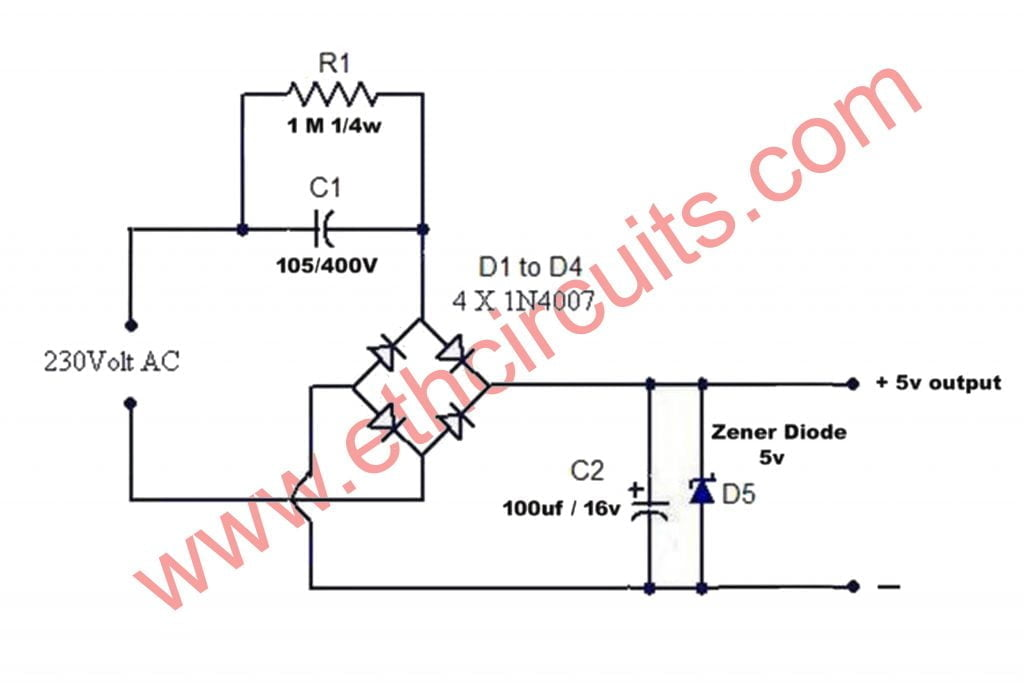# What Is Capacitor Power Supply ?

A capacitor power supply, also called a capacitive dropper, is a type of power supply that uses the capacitive reactance of a capacitor to reduce the mains voltage to a lower voltage.Capacitor Power Supply also Called As Transformerless power supply.

## Capacitor Power Supply Limitations

There are two important limitations:

First, the high withstanding voltage required of the capacitor, along with the high-capacitance required for a given output current, mean that this type of supply is only practical for low-power applications. (The capacitance needed increases with the current to be drawn; high capacitance mains-voltage capacitors are expensive and bulky.)

The second is that due to the absence of electrical isolation between input and output, anything connected to the power supply must be reliably insulated so that it is not possible for a person to come into electrical contact with it. By the equation of state for capacitance, where {\displaystyle I_{c}=C{\frac {\mathrm {d} V}{\mathrm {d} t}}}, the current is limited to: 1 amp, per farad, per volt-rms, per radian (of phase). Or {\displaystyle 2\pi }amps, per farad, per volt-rms, per hertz.

## Transformerless Power Supply Circuit Diagram## Parts List For Transformerless Power Supply Circuit

• Diode IN4007 4pc, D5 (Zener diode) 5v 1watt
• Capacitor 105/400v , 100uf/16v
• Resistor 1 mega

## Explanation Of Transformerless Power Supply Circuit

Hello friends this circuit related to capacitor power supply you can also say transformerless power supply circuit diagram its working perfectly .

You can change zener diode and change the voltage if you need 12 volt output use 12 volt zener diode instead 5 volt . This circuit gives 100 ma current . For 500 ma power supply circuit

error: Content is protected !!
Scroll to Top